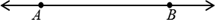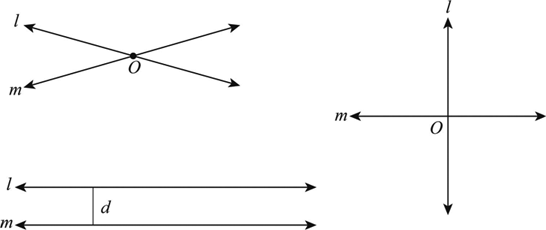Home/All Courses/CBSE/NCERT/NCERT Solutions For Class 9/NCERT Solution For Class 9 Maths

# NCERT Solution For Class 9 Maths

## NCERT Solutions for Class 9 Maths Chapter 15 – Probability

2018-10-31T12:36:23+00:00 Categories: NCERT Solution For Class 9 Maths||

Probability class 9 ncert solutions pdf covers the topic of Probability and probability formulas. To get in-depth knowledge on probability class 9 notes the students can refer to probability class 9 ncert solutions pdf given below.

## Probability

Probability is the measure of the likeliness that an event will occur. Probability is quantified as a number between 0 and 1 […]

## NCERT Solutions for Class 9 Maths Chapter 14 – Statistics

2018-10-31T12:00:51+00:00 Categories: NCERT Solution For Class 9 Maths||

NCERT solutions for class 9 maths chapter 14 pdf covers the topic of Collection of Data,  Presentation of Data, Graphical Representation of Data. The chapter also discusses about mean, median, mode. To get in-depth information and statistics class 9 notes you can refer to ncert solutions for class 9 maths chapter 14 pdf given below.

## Statistics

Statistics deals with collection, […]

## NCERT Solutions for Class 9 Maths Chapter 13 – Surface Areas and Volumes

2018-10-31T11:15:53+00:00 Categories: NCERT Solution For Class 9 Maths||

Ncert Solutions for Class 9 Maths Chapter 13 pdf covers the topic of surface areas and volumes class 9 and the formulas to calculate the areas and volumes of Square, Rectangle, Triangle, Circle. To get more in-depth knowledge on surface areas and volumes class 9 refer to  ncert solutions for class 9 maths chapter 13 […]

## NCERT Solutions for Class 9 Maths Chapter 12 – Heron’s Formula

2018-10-24T11:13:28+00:00 Categories: NCERT Solution For Class 9 Maths||

NCERT Solutions for Class 9 Maths Chapter 12 PDF covers the topic of triangles, different types of triangles and the formulas to calculate the areas of triangle. The chapter also discusses Herons formula and area of trapezoid

## Area of Triangle:

A triangle is a regular polygonal structure which has three sides. The sum of all angles is […]

## NCERT Solutions for Class 9 Maths Chapter 10 – Circles

2018-10-24T11:27:02+00:00 Categories: NCERT Solution For Class 9 Maths||

Circle, it is one of the basic shapes which we come across in our day to day life. The distance between the center of the circle and the edges remains the same from any given point on the edge. It is round in shape having a definite circumference. IN other words you can all a […]

## NCERT Solutions for Class 9 Maths Chapter 9 – Areas of Parallelograms and Triangles

2018-10-24T09:30:07+00:00 Categories: NCERT Solution For Class 9 Maths||

Class 9 Maths chapter 9 solutions pdf has a number of questions on area of triangle and area of parallelogram. Practice the solutions as many times as possible to master the chapter!

## Area of Triangle:

A triangle has three sides which are joined to each other. A triangle in which of the angle is 90 degrees […]

## NCERT Solutions for Class 9 Maths Chapter 8 – Quadrilaterals

2018-10-24T09:23:53+00:00 Categories: NCERT Solution For Class 9 Maths||

A Quadrilateral has four-sides, it is 2-dimensional (a flat shape), closed (the lines join up), and has straight sides.

• A quadrilateral has 4 sides, 4 angles and 4 vertices.
• A quadrilateral can be regular or irregular.
• The sum of all the interior angles of a quadrilateral is 360 degree.

## Properties of Parallelogram

There are six important properties of parallelograms to acknowledge:

[…]

## NCERT Solutions for Class 9 Maths Chapter 7 – Triangles

2018-10-24T11:39:43+00:00 Categories: NCERT Solution For Class 9 Maths||

A triangle has three sides and three angles. The three angles always add to 180 degree. There are three special names given to triangles that tell how many sides (or angles) are equal.

There can be 32 or no equal sides/angles:

## Equilateral

• Three equal sides
• Threeequal angles, always 60°

## Isosceles

• Two equal sides
• Two equal angles

## Scalene

• No equal sides
• No equal angles

Triangles […]

## NCERT Solutions for Class 9 Maths Chapter 6 – Lines and Angles

2018-10-24T09:15:38+00:00 Categories: NCERT Solution For Class 9 Maths||

## Line

A line has neither initial nor terminal points with indefinite length.The pairs of lines are nothing but the two lines, which may be intersects or parallel or perpendicular as shown below:The […]

## NCERT Solutions for Class 9 Maths Chapter 5 – Introduction to Euclid’s Geometry

2018-10-24T09:15:15+00:00 Categories: NCERT Solution For Class 9 Maths||

Euclidean geometry is the study of plane and solid figures on the basis of axioms and theorems employed by the Greek mathematician Euclid. In its rough outline, Euclidean geometry is the plane and solid geometry commonly taught in secondary schools. For details
class 9 maths chapter 5 solutions can be referred to.

## Euclid’s axioms

·         Things which […]

Hello